# PostgreSQL Tutorial: WHERE

August 1, 2023

Summary: in this tutorial, you will learn how to use PostgreSQL `WHERE` clause to filter rows returned by a `SELECT` statement.

The `SELECT` statement returns all rows from one or more columns in a table. To select rows that satisfy a specified condition, you use a `WHERE` clause.

## PostgreSQL `WHERE` clause overview

The syntax of the PostgreSQL `WHERE` clause is as follows:

``````SELECT select_list
FROM table_name
WHERE condition
ORDER BY sort_expression
``````

The `WHERE` clause appears right after the `FROM` clause of the `SELECT` statement. The `WHERE` clause uses the `condition` to filter the rows returned from the `SELECT` clause.

The `condition` must evaluate to true, false, or unknown. It can be a boolean expression or a combination of boolean expressions using the `AND` and `OR` operators.

The query returns only rows that satisfy the `condition` in the `WHERE` clause. In other words, only rows that cause the `condition` evaluates to true will be included in the result set.

PostgreSQL evaluates the `WHERE` clause after the `FROM` clause and before the `SELECT` and `ORDER BY` clause:If you use column aliases in the `SELECT` clause, you cannot use them in the `WHERE` clause.

Besides the `SELECT` statement, you can use the `WHERE` clause in the `UPDATE` and `DELETE` statement to specify rows to be updated or deleted.

To form the condition in the `WHERE` clause, you use comparison and logical operators:

Operator Description
= Equal
> Greater than
< Less than
>= Greater than or equal
<= Less than or equal
<> or != Not equal
AND Logical operator AND
OR Logical operator OR
IN Return true if a value matches any value in a list
BETWEEN Return true if a value is between a range of values
LIKE Return true if a value matches a pattern
IS NULL Return true if a value is NULL
NOT Negate the result of other operators

## PostgreSQL WHERE clause examples

Let’s practice with some examples of using the `WHERE` clause. We will use the `customer` table from the sample database for demonstration.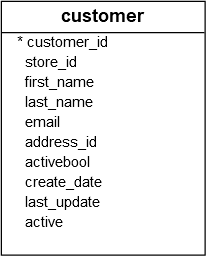### 1) Using WHERE clause with the equal (`=`) operator example

The following statement uses the `WHERE` clause to find customers whose first names are `Jamie`:

``````SELECT
last_name,
first_name
FROM
customer
WHERE
first_name = 'Jamie';
``````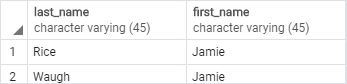### 2) Using WHERE clause with the `AND` operator example

The following example finds customers whose first name and last name are `Jamie` and `rice` by using the `AND` logical operator to combine two Boolean expressions:

``````SELECT
last_name,
first_name
FROM
customer
WHERE
first_name = 'Jamie' AND
last_name = 'Rice';
``````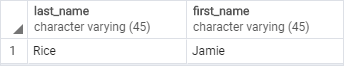### 3) Using the WHERE clause with the `OR` operator example

This example finds the customers whose last name is `Rodriguez` or first name is `Adam` by using the `OR` operator:

``````SELECT
first_name,
last_name
FROM
customer
WHERE
last_name = 'Rodriguez' OR
``````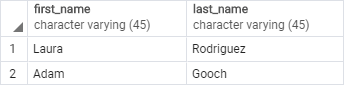### 4) Using WHERE clause with the `IN` operator example

If you want to match a string with any string in a list, you can use the `IN` operator.

For example, the following statement returns customers whose first name is `Ann`, or `Anne`, or `Annie`:

``````SELECT
first_name,
last_name
FROM
customer
WHERE
first_name IN ('Ann','Anne','Annie');
``````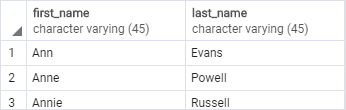### 5) Using the WHERE clause with the `LIKE` operator example

To find a string that matches a specified pattern, you use the `LIKE` operator. The following example returns all customers whose first names start with the string `Ann`:

``````SELECT
first_name,
last_name
FROM
customer
WHERE
first_name LIKE 'Ann%'
``````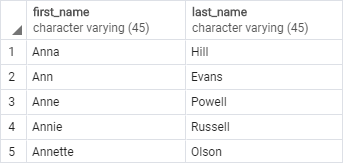The `%` is called a wildcard that matches any string. The `'Ann%'` pattern matches any string that starts with `'Ann'`.

### 6) Using the WHERE clause with the BETWEEN operator example

The following example finds customers whose first names start with the letter `A` and contains 3 to 5 characters by using the `BETWEEN` operator.

The `BETWEEN` operator returns true if a value is in a range of values.

``````SELECT
first_name,
LENGTH(first_name) name_length
FROM
customer
WHERE
first_name LIKE 'A%' AND
LENGTH(first_name) BETWEEN 3 AND 5
ORDER BY
name_length;
``````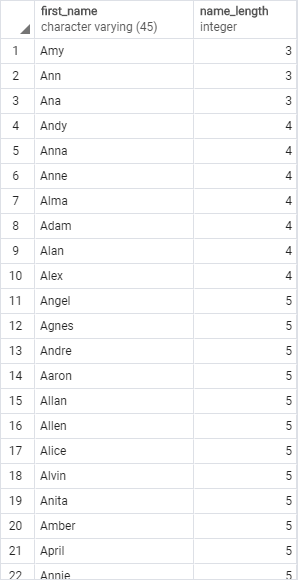In this example, we used the `LENGTH()` function gets the number of characters of an input string.

### 7) Using the WHERE clause with the not equal operator (<>) example

This example finds customers whose first names start with `Bra` and last names are not `Motley`:

``````SELECT
first_name,
last_name
FROM
customer
WHERE
first_name LIKE 'Bra%' AND
last_name <> 'Motley';
``````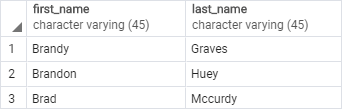Note that you can use the `!=` operator and `<>` operator interchangeably because they are equivalent.

In this tutorial, you have learned how to use PostgreSQL `WHERE` clause in the `SELECT` statement to filter rows based on a specified condition.